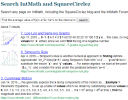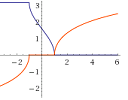# Posts tagged ‘Technology’

## How to search for solutions to math questionsA lot of students struggle to find answers for their math questions. Here are some helpful hints.

## IntMath Newsletter: Curvilinear, anchoring, pancakes

1. New on IntMath: Curvilinear motion animated graphs
2. Estimation and the anchoring principle
3. Bill Gates and his pancakes
4. Math movies
5. Math puzzle: Who dies?
6. Final thought: Truth

## IntMath Newsletter: Series, clock, Simpsons

1. New on IntMath: Infinite series, Spinning clock
2. Resources: Simpsons math, Unknowables
3. Math in the news: Plimpton 322
4. Math movies
5. Math puzzle: EFFEKT
6. Final thought: Actions

## IntMath Newsletter: Shells, resource, Bitcoin

1. New on IntMath: Shell method
2. Resource: Mathpix
3. Math in the news and Math movie: Bitcoin
4. Math puzzle: Triangles
5. Final thought: No more cars?

## What’s the correct graph of sec(arccos(x))?Here we solve another graph mystery - what is the correct domain for y = sec(arccos(x))? And why do different software packages show similar, but different graphs?

## IntMath Newsletter: trig differentiation applet, art, music

1. Differentiation applet - trigonometric functions
2. Math art
3. Resource: SimplexNumerica
4. Math movie: Music And Measure Theory
5. Math puzzles
6. Final thought: Challenges

## IntMath Newsletter: squaring circle, 17 equations

0. PhotoMath
1. Squaring the circle with rope
2. East Asian countries perform best in TIMMS
3. Resource: 17 equations
4. 2016 in review
5. Math movies: Unexpected math
6. Math puzzles
7. Final thought: tolerance

## IntMath Newsletter: hyperbolas, Pixar, PhotoMath

1. Interactive hyperbola graphs
2. Pixar in a box
3. Resource: PhotoMath
4. Math movie: Teach for Mastery
5. Math puzzles
6. Final thought: beliefs

## IntMath Newsletter: Angular velocity, face numbers, WIRIS

1. Linear & angular velocity applet
2. Your face in numbers: Microsoft's Face Detection app
3. Resource: WIRIS Calc
4. Math movie: Conspiracy theories & math
5. Math puzzles
6. Final thought